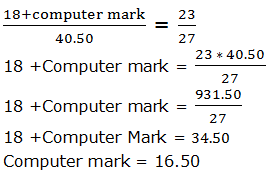# Missing DI Practice Set for RBI Grade B Part-2

(Direction 1 -5) Following is a tabulation of marks scored by five students in IBPS PO mains exam with some missing data. Read the table carefully and answer the questions.

Name Aptitude
(50)
Reasoning
(50)
English
(40)
GA
(40)
Computer
(20)
Overall Score (200)
Arjun - 27.00 20.75 30.25 15.25 118.75
Bipin 22.75 - 15.50 - 14.75 98.75
Chandana - 26.75 - 15.25 17.50 -
Dwarkesh 35.50 30.25 - - 18.00 124.25
Eman - 22.50 17.25 22.75 - -

1. What is the score of Chandana in English, if overall percentage she has scored is 50.125 and scored three marks more than GA section in Aptitude section?
A.20.50
B. 21.50
C. 22.50
D. 23.50
E. None of these

2. If mark scored by Bipin in GA and mark scored by Dwarkesh in English are equal and If the difference between English and GA marks of Dwarkesh is 0.50, then How many of them have scored more than 50 % marks in Reasoning Section?
A. 5
B. 4
C. 3
D. 2
E. None of these

3. If the ratio between computer marks scored by Dwarkesh and Eman to English and GA marks scored by Dwarkesh is 27:23, then what will be the mark scored by Eman in Computer section?
A. 16.50
B. 16.75
C. 15.50
D. 15.75
E. None of these

4. Who have scored maximum mark in Aptitude section, if the sum of aptitude mark of Arjun and Chandana is 43.75, which is 12.50 marks more than Eman mark in aptitude?
A. Arjun
B. Bipin
C. Eman
D. Dwarkesh
E. None of these

5. What is the minimum score required by Eman in aptitude section to clear the overall cut off 90.50, if he has scored 55% mark in computer section?
A. 17.00
B. 17.25
C. 17.50
D. Cannot be determined
E. None of these

#### 1. Option C

Overall score= (50.125/100) x 200 = 100.25
Aptitude score = 15.25 +3 = 18.25
English score = 100.25 – ( 18.25+26.75+15.25+17.50)
(100.25 – 77.75)= 22.50

#### 2. Option B

• Sum of English and GA mark of Dwarkesh = 124- (35.50+30.25+18.00)
• 40.50
• Since Difference between English and GA marks is 0 .50, Individual marks will be 20.00 and 20.50
• Therefore GA mark of Bipin will be either 20.00 or 20.50
• If GA mark of Bipin is 20.00, then his reasoning mark will be 25.75
• If GA mark of Bipin is20.50 , then his reasoning mark will be 25.25
• Therefore Reasoning mark of Bipin will be more than 50%
• Students who have scored more than 50% marks in reasoning are Arjun, Bipin, Chandana and Dwarkesh

#### 3. Option A#### 4. Option D

Aptitude mark of Arjun = 118.75 - (27.00+ 20.75+ 30.25+ 15.25) = 25.50
Aptitude mark of Bipin = 22.75
Aptitude mark of Chandana = 43.75 – 25.50 = 18.25
Aptitude mark of Dwarkesh = 35.50
Aptitude mark of Eman = 43.75- 12.50 = 31.25
So, Dwarkesh scores the highest mark in aptitude.

#### 5. Option A

Computer mark of Eman = (55/100) x 20 = 11
Min. mark required in aptitude sec = 90.50-(22.50+17.25+22.75+11) =17.00